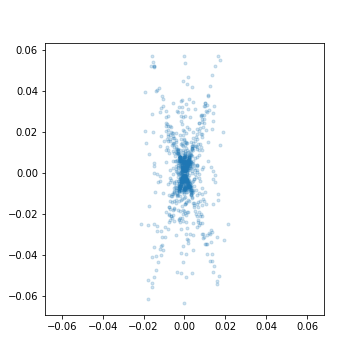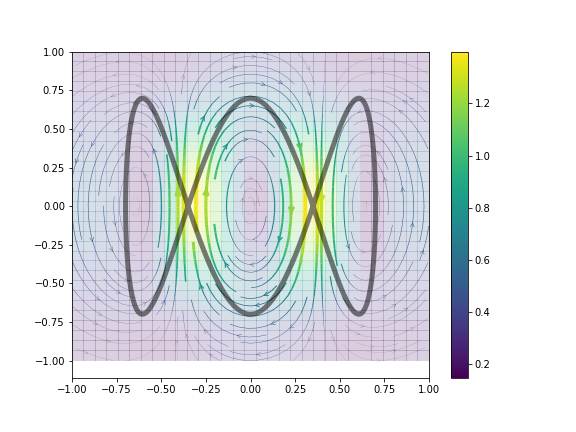# olivierverdier/femshape

Library for computing shape invariants of planar curves using finite element methodFetching latest commit…
Cannot retrieve the latest commit at this time.
Type Name Latest commit message Commit time
Failed to load latest commit information.README.md__init__.pyfemshape.pyinvariants.pngmonomials.pypca.pyrepresenter.pngrun_invars.ipynbrunit.pyseparate_shapes.ipynbshape_test.ipynbutils.py

This is a Python library for computing shape invariants of planar curves using FEM and the FEniCS package. To run the module, FEniCS must be installed.

It is the supporting code for the paper Currents and finite elements as tools for shape space by James Benn, Stephen Marsland, Robert I McLachlan, Klas Modin and Olivier Verdier.

## Getting started

The simplest way to calculate the invariants of a curve are as follows.

1. Create a space
`space = Space()`

Check the documentation of the `Space` class to see the available options.

1. Create a curve

This is just an arbitrary array of size P x 2, where P is the number of points. For instance,

```ts = np.arange(0, 2*np.pi, 200)
curve = .7*np.array([np.cos(ts), np.sin(3*ts)]).T```
1. Compute the associated current
`current = Current(space, curve)`

You have now access to the property `invariants` which contains the result of the current evaluated on the basis of one-forms.

You can inspect the invariants using

`plot_invariants(current)`1. Compute the representer

Givne an underlying Hilbert space structure on the one forms, one can compute the associated representer of the current:

`representer = Representer(current, scale=.2)`

You can plot the result using

`plot_representer(representer)`## Further examples

The notebooks contain further examples:

You can’t perform that action at this time.